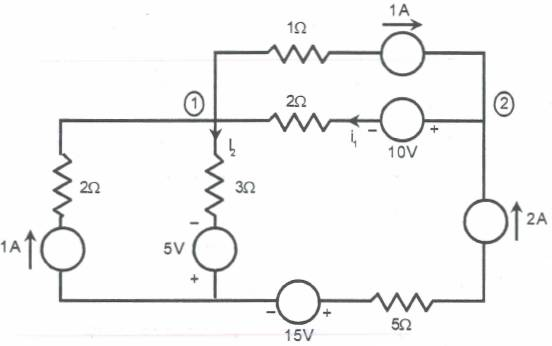# Problem with Node Voltage Method

1. Find the power delivered by the 5V Voltage source in the network of Fig.This is my attept at solution:

At node 1 V1...assuming current leaving V1:
KCL equation: -1A+1A+[(V1-5)/3]+[(V1-(V2-10))/2]=0

At node 2 V2...assuming current leaving V2:
KCL equation: -2A-1A+[V2-(V1+10)]=0

But, I'm not certain about the sign convention when there is a voltage source with a resistor between V1 and V2....
I'm also confused that how to proceed when there is a current source and resistor between two nodes.....
Where did I go wrong...?

Last edited:

gneill
Mentor
If you look at the node labelled 2, you'll note that three branches come together and two of them have specified currents. What does KCL tell you about the current in the remaining branch? Can you now repeat this feat at node 1?

If you look at the node labelled 2, you'll note that three branches come together and two of them have specified currents. What does KCL tell you about the current in the remaining branch? Can you now repeat this feat at node 1?
Thank you
Yes, there are four branches coming together at node 1.Currents are specified for two of the branches.

At node 1 KCL: -1+1+[(v1-(-5))/3]+[v1-(v2+10)]/2=0

Did I write it correctly?
Thanks...

gneill
Mentor
Thank you
Yes, there are four branches coming together at node 1.Currents are specified for two of the branches.

At node 1 KCL: -1+1+[(v1-(-5))/3]+[v1-(v2+10)]/2=0

Did I write it correctly?
Thanks...

You won't even need the node voltage... If you know all but one current
flowing through the node, the last one is thus determined y the others.
So what must i2 be?

I think i2=(v1+5)/2, am I correct?

gneill
Mentor
Look again at node 1. You've determined what i1 must be, coming from node 2, right? So all branches leading to node 1 have known currents except for the branch with i2. So what's i2?

As 2A and 1A are reaching node 2....3A must leave it..

So, i1=3A, am I correct..?
and wow i2=3A...I got it now ....I think I'm correct...amn't I?
Thank you very very much...

Last edited:
gneill
Mentor
As 2A and 1A are reaching node 2....3A must leave it..

So, i1=3A, am I correct..?
and wow i2=3A...I got it now ....I think I'm correct...amn't I?
Thank you very very much...

Yup.Now you can figure out the power delivered by the 5V supply.